# Struggling to Get Triangles Homework Answers Online? Try AnswerAddict Triangle Homework Experts!Do you have a kid in the elementary level who struggles to understand triangle concepts or write triangle homework? Read along to learn insightful tips about triangle worksheets and triangle homework and how best a student can succeed.

Even though the triangle concept is pretty basic and seems like a walkover for most students, we cannot ignore kids who are just getting started—because we all trace our roots back there. It is always challenging to get started, and for the most part, you need a teacher or tutor to take your child through everything stepwise until they understand everything about triangles.

First, we must acknowledge that elementary school teachers give their all to support students in understanding and solving different problems. But we should not also cast a blind eye on the possible online homework resources that students can utilize while at home to streamline their learning curve.

At AnswerAddicts, we have the best online math tutors who offer quality triangle homework help. So, we aim to enlighten you about the most fundamental concepts of triangles to ensure your child gets all the basics about triangles.

### Stormed with Homework? Get professional homework help today## What Is a Triangle?

A triangle is the simplest form of polygons and is made up of three sides joined end to end. So by extension, it has three angles. To understand it better, break it down into two words—"tri," which means three, and "angle." Therefore, a triangle three sides, three angles, three vertices, and the sum of interior angles are equal to 180°.

## The 5 Basic Features of a Triangle

The most fundamental properties of a triangle include:

• Triangles are made up of three sides, vertices and angles.
• According to the "Angle Sum Property" of angles, the sum of all interior angles of a triangle is 180°.
• If you sum up the lengths of two sides of any triangle, the resultant length must be greater than the length of the third side.
• The longest side of a triangle is that which is opposite to the largest angle of a triangle.
• According to the exterior angle property of triangles, any exterior angle is equal to the sum of the two interior angles opposite to the interior angle.

## The Geometry Triangle Worksheet on Types of Angles

The different types of angles in a triangle are:

Acute angle

Right angle

Obtuse angle

Straight angle

Reflex angle

Complete angle

Let us discuss each in detail.

### Acute Angle

An acute angle is usually less than 90°. Typical examples that you will often come around with include 15°, 30°, 45°, and 60°. Check out the illustration below.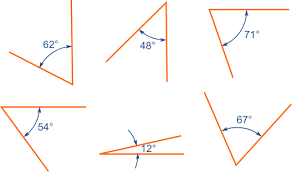### Right Angle

Right angles measure exactly 90°. You will meet this feature on various applications, especially if you hope to get into Physics and Engineering. The 90° results from the intersection of two perpendicular lines. When the two intersecting lines are equal, the resultant opposite angles are equal—45°. And such triangles are referred to as congruent triangles.

However, if the two sides are not equal, then the resultant triangle is called a scalene right triangle, which has one right angle triangle and two angles or different sizes. A good example of two such angle pairs is 60° and 30°.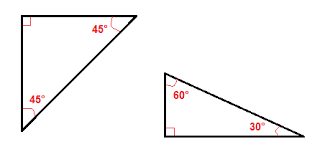### Obtuse Angle

An obtuse angle measures between 90° and 180°. Therefore, it is greater than a right angle triangle but less than a reflex angle, as we shall see shortly. The commonest obtuse angles you can expect to come across include 100°, 120°, and 150°.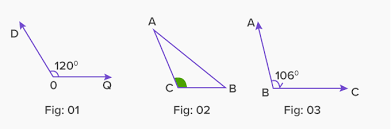### Straight Angle

Any point along a straight line is a straight angle, which means it measures 180°. A practical example of a straight-angle geometry is an angle formed in a see-saw or a plane inclined staircase. Here is a typical representation.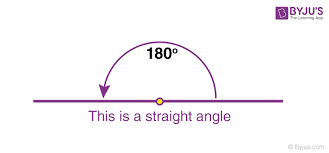### Reflex Angle

A reflex angle in triangle worksheets measures between 180° and 360°. In geometry problems, reflex angles are usually external angles because the angle sum of a triangle is 180°.### Complete Angle

As the name suggests, a complete angle is an angle whose measure is exactly 360°. It is also called a turn, revolution, complete circle, or a cycle. It is represented as shown below: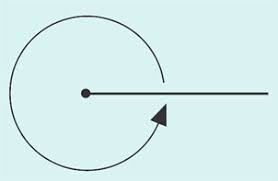## Triangle Worksheets- the Different Types of Triangles

We can classify triangles from two perspectives:

• Based on internal angles
• Based on the length of triangle sides

### How To Classify Triangles Based on Internal Angles

• Acute Angled Triangle
• Right-Angled Triangle
• Obtuse Angled Triangle

Now let us dive into each and discuss them in detail.

1. Acute Angled Triangle

As the name suggests, acute-angled triangles are those in which all the three internal triangles are acute—less than 90°. Remember that we mentioned that the sum of all interior angles should add up to 180°. For instance, when asked to draw an acute angle triangle, the answer can be something like: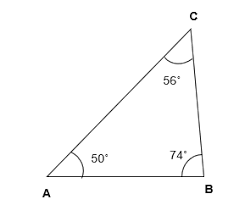2. Right-Angled Triangle

True to its name, one of the angles in a right-angled triangle is 90°. The edge/side opposite the right angle is the longest—also called the hypotenuse. As we mentioned earlier, it becomes a congruent/isosceles triangle if the other two sides have equal lengths and scalene if they are different.

Example of a congruent right-angled triangle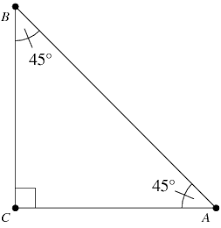Example of a scalene right-angled triangle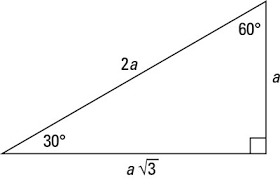3. Obtuse Angled Triangle

One of the angles in an obtuse triangle is greater than 90° but less than 180°. In a nutshell, it is named after the angle. Questions around this type of triangle may require you to calculate the size of the other interior angles and the exterior angles—using different identities in geometry.

An example of an obtuse-angled triangle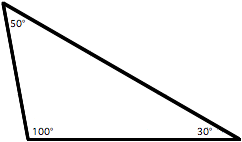### How To Classify Triangles Based on the Length of their Sides?

• Equilateral Triangle
• Isosceles Triangle
• Scalene Triangle

#### Equilateral Triangle

All the sides in an equilateral triangle are equal. That translates to having equal interior angles, all of which measure 60°. It is also one of the perfectly congruent triangles in geometry because all sides and angles are equal.

Example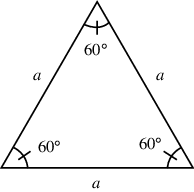#### Isosceles Triangle

An isosceles triangle in geometry is one in which two sides are equal, which translates to two equal interior angles. In most cases, you will be given one of the angles—either the 'odd' one or one of the congruent angles. Then you are required to calculate the other angles. Since you know the total sum should be 180°, it is pretty straightforward to calculate the other angles.

Here is an illustration: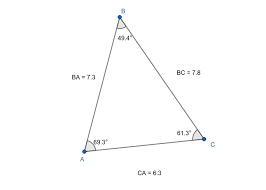#### Scalene Triangle

In geometry, scalene triangles are those that have unique edge lengths. That means all the interior and exterior angles are also unique, which means they do not have a line of symmetry.

Example of a scalene triangle### Stormed with Homework? Get professional homework help today## Way Forward: How Do You Succeed in Solving Math Problems in Triangles?

If you are finding challenges with questions on triangles, there are a few tips you can embrace today and see yourself succeed.

Here is what to do:

Commit yourself to learn and understand the basic concepts we have shared in this triangle worksheet. Well, they may sound simple now, but if you do not understand each of them and their related features, you will find just how confusing they are in the exam room. Take each new concept, analyze it, then dig deep into its advanced concepts.

Learn related concepts: Reinforce what you have learned with related concepts such as area and perimeter. That is because most questions are usually tied. You need this knowledge to score a good grade in math.

Practice: Math has no other secret. If you want to score a good grade, then you must be willing to pay the price. And the price is simply committing yourself to do as many questions as possible. That exposes you to the tricks that examiners keep testing to ensure you do not have a hard time with your triangle assignments or exams.

## Are Triangle Questions Challenging? We Offer Solutions!

You may go through as many triangle worksheets as possible but still find that challenging problem that not even your classmates can do. In that case, do not wait to fail. Answeraddict online tutors are always available 24/7 to ensure every student gets solutions to their disturbing problems.

The good thing is, we do all that at almost free rates because we understand these are pretty basic concepts, so they should cost an arm and a leg. Place an order or send us a message so we can discuss how we can help you.